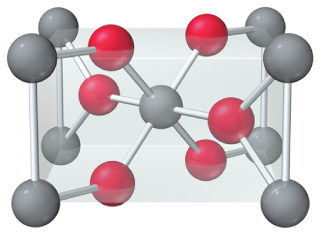# Problem: An oxide of titanium crystallizes with the following unit cell (titanium = gray; oxygen = red).What is the formula of the oxide?

###### FREE Expert Solution

There are 9 titanium atoms in the structure of which eight are at the corners of the unit cell and one in the center.

Contribution of one atom at the corner: 1/8

There are 8 atoms hence

$\mathbf{8}\mathbf{×}\frac{\mathbf{1}}{\mathbf{8}}\mathbf{=}$1

Contribution of 1 atom at the center: 1

Total number titanium atoms: 2

There are 6 oxygen atoms in the structure, four of which are at the face centers and two are close to the center.

In face centers, the contribution is 1/2

Therefore the number of oxygen atoms is:

$\left(2×1\right)\mathbf{+}\left(4×\frac{1}{2}\right)\mathbf{=}$4

93% (94 ratings)###### Problem Details

An oxide of titanium crystallizes with the following unit cell (titanium = gray; oxygen = red).

What is the formula of the oxide?